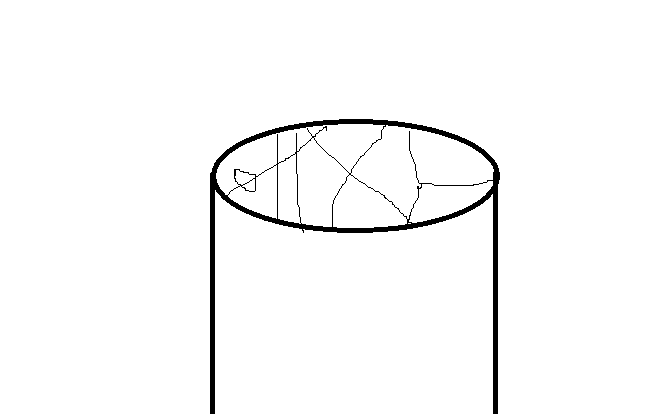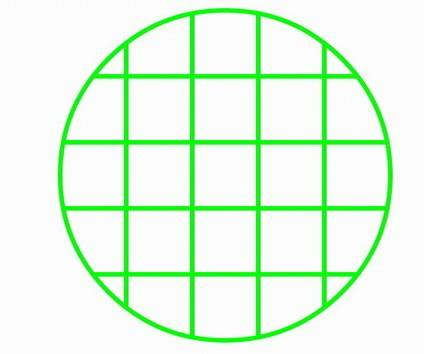# How to divide a cylinder

hello

I have a cylinder

which and how many lines will I have to draw in its base to delimit holes that will run through it, so that I will maximize its internal surface?

thanks!

Mark44
Mentor
hello

I have a cylinder

which and how many lines will I have to draw in its base to delimit holes that will run through it, so that I will maximize its internal surface?

thanks!

why you say so?
what else you need to know?
you can take a cylinder 10cm diameter, if that's what you need to know
I need to find the optimum diameter of the holes and the highest number of holes
but holes may not be circular, they can be any shape

you can do it for a circle, how many shapes you can draw in it to maximize the length of the lines in it
and then we can integrate it

Mark44
Mentor
why you say so?
what else you need to know?
How is the cylinder oriented? Is it horizontal or vertical?

which and how many lines will I have to draw in its base to delimit holes that will run through it, so that I will maximize its internal surface?
I don't understand what this means. How do lines drawn in its base delimit holes running through it? What does this have to do with maximizing its internal surface?

These are some of the questions that came to mind when I said that your question was too vague to answer.

what you mean how the cylinder is oriented? it doesn't matter, you just have a cylinder

when you draw lines in the cylinder's base, you delimit holes, right? do you understand this? holes that run the cylinder across its length

Mark44
Mentor
what you mean how the cylinder is oriented? it doesn't matter, you just have a cylinder
This would make a difference if you filled the cylinder with some liquid. I thought that might be what you were after.
physior said:
when you draw lines in the cylinder's base, you delimit holes, right? do you understand this? holes that run the cylinder across its length
No, I don't understand this. If I have a circular cardboard cylinder, with circular top and bottom caps, I can use a pen to draw lines on the end caps. I don't see how these lines have anything to do with holes in the cylinder wall.

A picture would be most helpful...

This would make a difference if you filled the cylinder with some liquid. I thought that might be what you were after.

yes, I may fill the cylinder with some liquid, but the orientation will have no effect at all, as the whole cylinder will be filled and not part of it, so that gravity and orientation could play a role, this is what you are saying? no, orientation doesn't matter

No, I don't understand this. If I have a circular cardboard cylinder, with circular top and bottom caps, I can use a pen to draw lines on the end caps. I don't see how these lines have anything to do with holes in the cylinder wall.

well it's simple
the lines you will draw are the walls of the holes, I said the lines delimit holes, isn't it simple to understand?

A picture would be most helpful...

here it is:just imagine holes across the cylinder with walls as the lines you draw on the base

ok, then, something more realistic please

The lines you have in post 7 are strange. I do not see where the holes are.

The lines you have in post 7 are strange. I do not see where the holes are.

yes, they are strange
aren't there holes with strange lines?
the holes are the white beween closed loops of these lines and the lines are the walls of the holes
the holes as I numerous times said, are running through the cylinder across its length
geez

jbriggs444
Homework Helper
yes, they are strange
aren't there holes with strange lines?
the holes are the white beween closed loops of these lines and the lines are the walls of the holes
the holes as I numerous times said, are running through the cylinder across its length
geez
I see only three closed loops in that picture. They overlap.

I see only three closed loops in that picture. They overlap.
you must not be serious...
check again there are many more...
closed is a loop if it closes with the external margins of the cylinder base too

jbriggs444
Homework Helper
you must not be serious...
check again there are many more...
closed is a loop if it closes with the external margins of the cylinder base too
No. A line that touches the wall is not a hole. That's a gap on the exterior of the cylinder.

Edit: In any case, if you count the walls as possible boundaries for loops then the "holes" you have include the entirety of the cylinder. There would be nothing left.

No. A line that touches the wall is not a hole. That's a gap on the exterior of the cylinder.

Edit: In any case, if you count the walls as possible boundaries for loops then the "holes" you have include the entirety of the cylinder. There would be nothing left.
is this some kind of joke?

anyway, here is what I am after:
see below these square areas in the circlehow can I maximise the total perimeter of these sub-areas of the circle?
simple question
can anyone do it?
what's the maths behind it?

jbriggs444
Homework Helper
is this some kind of joke?

anyway, here is what I am after:
see below these square areas in the circlehow can I maximise the total perimeter of these sub-areas of the circle?
simple question
can anyone do it?
what's the maths behind it?
Which sub areas? Just the square ones? Do we get to double-count the perimeter of four unit squares plus the perimeter of the two by two square that contains them? What about a 2 by 3 rectangular area? What constraints do we have on the number of lines that can be used?

no constrains
don't overlap areas
do you even have the slightest idea of how to do the maths?

jbriggs444
Homework Helper
no constrains
don't overlap areas
do you even have the slightest idea of how to do the maths?
Subject to no constraints... there is no maximum total perimeter. Just draw lines in a grid as fine as you please.

The personal attacks are not appreciated.

ok then is there a preference of how the lines should be drawn to maximize the perimeter of the areas for a 10cm diameter cylinder and minimum 0.01mm space of area?

jbriggs444
Homework Helper
Still restricting our attention to a rectangular grid with straight lines but requiring a separation of 0.01 mm between each line? That's rather trivial. You are after an approximate formula for the achievable perimeter or a prescription for how to achieve it?

I am after a mathematical proof that the optimum way is for example to divide the circle into smaller circles, or squares, or triangles, or a combination, or whatever

can that be done?

jbriggs444
Homework Helper
I am after a mathematical proof that the optimum way is for example to divide the circle into smaller circles, or squares, or triangles, or a combination, or whatever

can that be done?
You have to clarify the constraints we are operating under. For a rectangular grid, you were willing to offer the constraint that the lines could be no closer the 0.01 mm apart. But that constraint does not translate directly to a different tessellation.

To come up with an "optimum" you need two things:

1. A valuation function so that one can compare possible solutions. Apparently we are taking total perimeter of the individual figures in a tessellation as our valuation function.

2. The constraints or rules that must be adhered to. That would include things like a requirement to use straight lines, convex figures, minimum line spacing and the like. If one is not told the rules of the game, it is difficult to come up with the best strategy.

I don't know about a valuation function

there are no constrains basically, apart from the fact that the small areas must be 0.01mm^2

jbriggs444